# Adapting a mechanical scenario to an accelerating reference frame near the surface of the earth

This article describes how, given an analysis of a mechanical scenario in the reference frame of the earth (for bodies near its surface), the same analysis can be adapted to an accelerating reference frame near the surface of the earth.

## Vertically accelerating reference frame

An example of this is an elevator (lift) that is accelerating up or down. Note that what matters is the direction and magnitude of acceleration, not that of velocity. For instance:

• When an elevator starts moving upward from a stationary position, its acceleration is upward. When an elevator originally moving upward loses speed in order to halt, its acceleration is downward.
• When an elevator starts moving downward from a stationary position, its acceleration is downward. When an elevator originally moving downward loses speed in order to halt, its acceleration is upward.

Suppose we want to perform analysis in such a reference frame (i.e., speeds and accelerations are measured relative to the elevator, so a person standing still in the elevator is considered to have zero acceleration). Here is how we can adapt former analyses:

• If the acceleration of the elevator is$a$ downward, we consider a pseudo-force of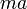$ma$ upward acting on each object of mass$m$. An effective way of incorporating this is to replace the original acceleration due to gravity$g$ by the value$g - a$. In particular, if the elevator is in free fall, then the acceleration due to gravity gets replaced by zero.
• If the acceleration of the elevator is$a$ upward, we consider a pseudo-force of$ma$ downward acting on each object of mass$m$. An effective way of incorporating this is to replace the original acceleration due to gravity$g$ by the value$g + a$. In particular, if the elevator is in free fall, then the acceleration due to gravity gets replaced by zero.

Here are some examples of conclusions drawn for ordinary mechanics scenarios and the analogous conclusions in elevators accelerating downward and upward:

Original scenario Analogous elevator scenario Original conclusion Conclusion for elevator (lift) with downward acceleration$a$ Conclusion for elevator (lift) with upward acceleration$a$
sliding motion along a frictionless inclined plane making an angle$\theta$ with the horizontal, where the inclined plane is fixed to the ground. sliding motion along a frictionless inclined plane, where the inclined plane is fixed to the elevator. Acceleration down the inclined plane is$g \sin \theta$ Acceleration down the inclined plane is$(g - a) \sin \theta$ Acceleration down the inclined plane is$(g + a)\sin \theta$.
sliding motion along an inclined plane (same as previous, but now there could be friction. Static and kinetic friction coefficients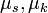$\mu_s, \mu_k$ respectively.) The inclined plane is fixed to the elevator rather than the ground. angle of repose is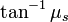$\tan^{-1} \mu_s$ -- this is the smallest angle at which sliding begins spontaneously
If sliding downward, acceleration downward is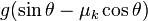$g(\sin \theta - \mu_k\cos \theta)$
angle of repose is still$\tan^{-1} \mu_s$
If sliding downward, acceleration downward is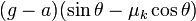$(g - a)(\sin \theta - \mu_k\cos \theta)$.
angle of repose is still$\tan^{-1} \mu_s$
If sliding downward, acceleration is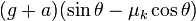$(g + a)(\sin \theta - \mu_k\cos \theta)$
pendulum in simple harmonic motion (sufficiently small amplitude of oscillations). End of pendulum is fixed relative to the ground. End of pendulum is fixed relative to the elevator. Time period is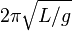$2\pi \sqrt{L/g}$ where$L$ is the length of the pendulum Time period is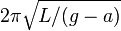$2\pi \sqrt{L/(g - a)}$ Time period is$2\pi \sqrt{L/(g + a)}$

## Horizontally or horizontally-cum-vertically accelerating reference frame

Examples of this would be vehicles (cars, ships, planes, etc.) that are accelerating in a particular horizontal direction). For such reference frames, we can use the same trick as before, but with a twist. We need to replace acceleration due to gravity$g$ by a new value, but we also need to change the direction of this acceleration. Specifically, we need to replace acceleration due to gravity by a vector sum of a downward vector for acceleration due to gravity, and the opposite vector to the acceleration vector.

For instance, if the reference frame has an eastward acceleration of$a$, we must replace acceleration due to gravity$g$ by the value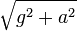$\sqrt{g^2 + a^2}$ and it points in a downward-cum-westerly direction, at an angle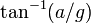$\tan^{-1}(a/g)$ with the vertical.

The effect is that the very notion of downward is different in a reference frame that has some horizontal acceleration.

To perform analysis in such a reference frame, then, it is necessary not only to change the magnitude of acceleration due to gravity but also to re-measure angles so that any previously measured angle with the vertical is replaced by the angle with the new downward direction.